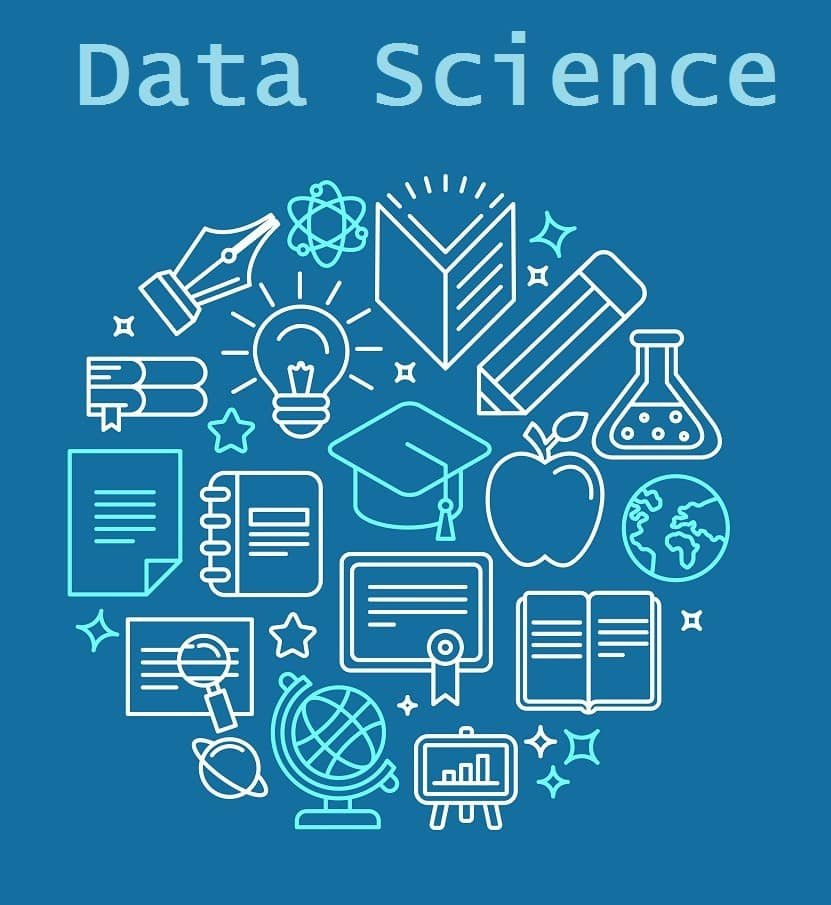# Data Science Quiz

0
859Try this Data Science Quiz to check how updated you are in the tech world. Go on and happy quizzing!! 🙂1.  ________ is not an activation function in neural networks. Fill in the blank.

2.  R is technically much closer to the Scheme language than it is to the which original language?

3. You’re a professor teaching Data Science with Python, and you want to visually assess if the grades (A, A-, B+, etc.) on your exam follow a particular distribution. Which plot do you use?

5. In computing and electronic systems, the binary-coded decimal is a class of binary encodings of decimal numbers where each decimal digit is represented by a fixed number of bits, usually four or eight. Convert 9 from decimal to binary.

6. ASCII is an acronym for ______

Answer – American Standard Code for Information Interchange

7. The command “apply()” applies a function over the ______ of an array. Fill in the blank.

8. What is the output of the following program: print(“Hello World”[::-1])?

9. An API is a computing interface that defines interactions between multiple software intermediaries. What is the full form of API?